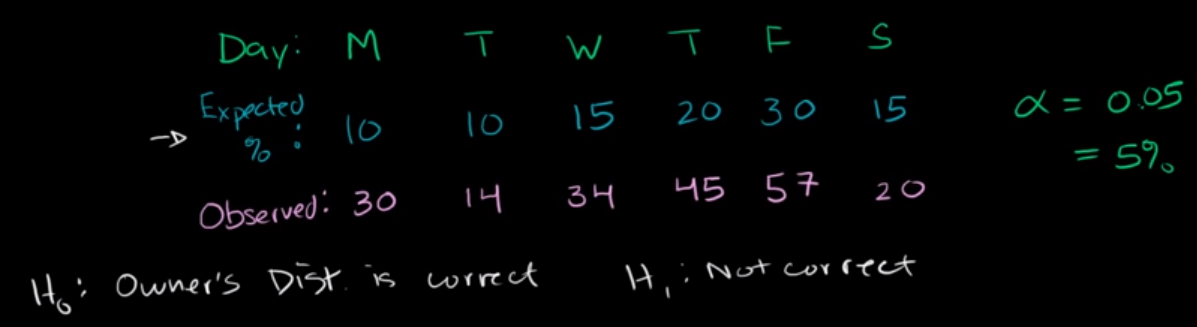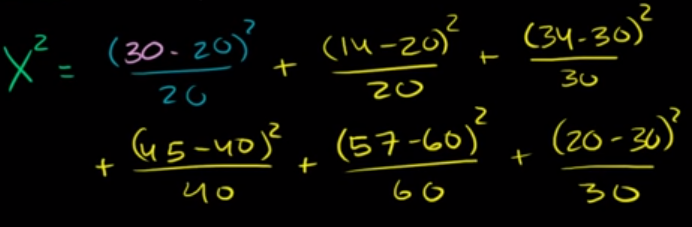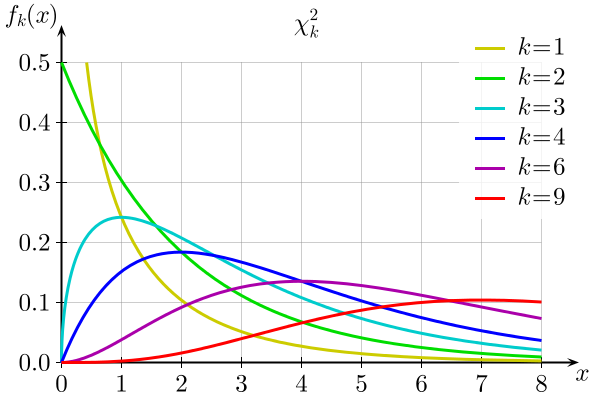# Chi Squared Goodness of Fit

## A Test for Comparing Discrete Distributions

I found Sal Khan’s explanation to be as straight-forward as it was useful.

Essentially, the Chi-Squared test is used when:

• You’ve got k discrete classes
• Some idea of their distribution
• A number of observed values that fall into said classes

In the case of the video, we want to check if a shop owner’s approximation of visitors-by-day as a percentage of their week is accurate or not.

from IPython.display import Image
Image('../images/chi_sq_ex.png')To solve a problem like this, we first want to rescale the total count of observed visitors (200) in a given week by the expected % value above, giving

20 20 30 40 60 30

Then, similar to how we might investigate a single normal distribution for each of these, we want to find the squared distance from observation to expected, normalized by expected.

The sum of these make up our Chi-Squared distribution, where our degrees of freedom is equal to the number of classes, minus 1– so 5.

Image('../images/chi_sq_ex2.png')Then, like any other test, we calculate the statistic and let a computer tell us how that translates into a p-value.

## The Distribution Itself

Borrowing jbstatistics’ explanation:

• If Z is standard normal, then Z-squared has a Chi-squared distribution, with one degree of freedom
• If Z1, ..., Zk are independent standard normals, then Z1^2 + ... + Zk^2 has a Chi-squared distribution with k degrees of freedom

These distributions take on different shapes, depending on how many Z terms you mix in (and by extension value of k).

Per wikipedia:

Image('../images/chi_sq_dist.png')Note: Each curve has:

• Mean: degrees of freedom
• Variance: degrees of freedom, squared
• Mode: degrees of freedom - 2

## Chi-Squared Tests in Python

Rehashing Sal’s example above, we’ll start with an array of the expected proportions, which sum to 1

import numpy as np

exp_prop = np.array([.10, .10, .16, .20, .30, .15])

Then we’ll create an array of our observed values

y_obs = np.array([30, 14, 34, 45, 57, 20])

Then, we’ll create an array, y_exp, that gives us the class count we’d expect to see, given the total count of observed people

y_exp = y_obs.sum() * exp_prop

Finally, performing the test is as easy as invoking a clean scipy.stats function

from scipy.stats import chisquare

chisquare(f_obs=y_obs, f_exp=y_exp)
Power_divergenceResult(statistic=11.033333333333333, pvalue=0.05072307251654765)